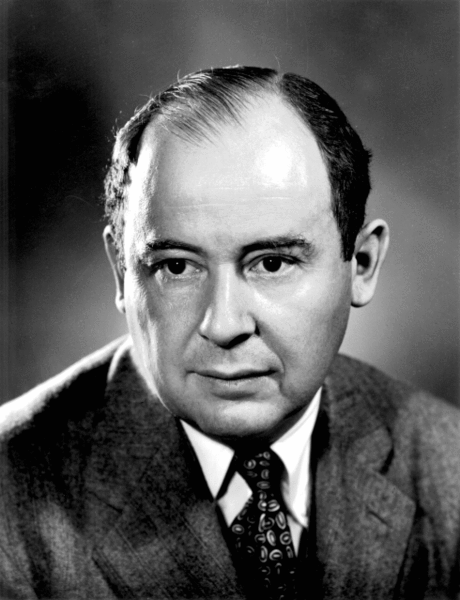# Von Neumann HierarchyJohn von Neumann

Set theory is so central and so fundamental to modern mathematics that it is easy to forget that little more than a century ago it seemed—even to most professional mathematicians—a mere diversion. To many it was quite suspect too, given that it treated infinities as respectable mathematical objects, something which had been taboo since Aristotle.

One of the mathematicians who showed how set theory may be taken as the very foundation of mathematics was John von Neumann (1903–1957). In the 1920’s he demonstrated how even the most basic objects in mathematics—the set of natural numbers—could be completely understood in terms of pure sets.

The construction is called the Von Neumann Hierarchy, and goes like this. Recall that sets can contain other sets as elements, and that the empty set is the set with nothing in it. We begin the construction with the empty set, and then at each step we form the set that contains all the previously constructed sets as elements.Figure 1: Starting the construction.

This goes on, of course, forever. If you take the entire (infinite) collection of all such sets and make a collection of them (a set!), then you have the Von Neumann Hierarchy of finite ordinals.

Keeping track of all those braces soon becomes impossible for the eye, so let’s adopt the convention of denoting the empty set by 0. We’ll denote the set $$\{0\}$$ containing the empty set by 1. So $$1=\{0\}$$. The next set, $$\{0,1\}$$, we’ll of course denote by 2. We get $$2=\{0,1\}$$, $$3=\{0,1,2\}$$, and so on. In general, given any natural number $$n$$, we have

$n+1 = \{0,1,2,3,4,\ldots,n\}$

In short, each ordinal is the set containing all lesser ordinals. (We might also have said that each successor ordinal is the power set of the previous ordinal—the definitions are equivalent.)

By letting the empty set represent 0 and defining successors in this way, we find that we have built a model of the Peano Axioms, which means we may define arithmetic on the finite ordinals by applying those axioms. And so, using pure sets, we have actually built the natural numbers—an astounding thing to do.

But we needn’t stop there; for there is nothing to prevent us considering the entire set of finite ordinals and taking it’s power set. This we will also call an ordinal—a limit ordinal rather than a successor ordinal—and we denote it by $$\omega$$. It is the first transfinite ordinal. We may then continue as before, defining $$\omega + 1$$ as $$\{\omega\}$$. Using the Peano Axioms we can even define $$\omega + \omega$$, $$\omega\cdot\omega$$, $$\omega^{\omega}$$, and so on. And then we can take the set of all such transfinite ordinals and (you guessed it) make a new limit ordinal out of them.

The entire universe of sets that is constructible in this way is called the Von Neumann Universe (of course), and it is the proper class of hereditarily well-founded sets. It is in considering the nature of such classes that the real work of researchers in set theory consists. The beginning student of mathematics should not shy away from thinking about such things for fear that they are too hard. It is a rich and rewarding field.